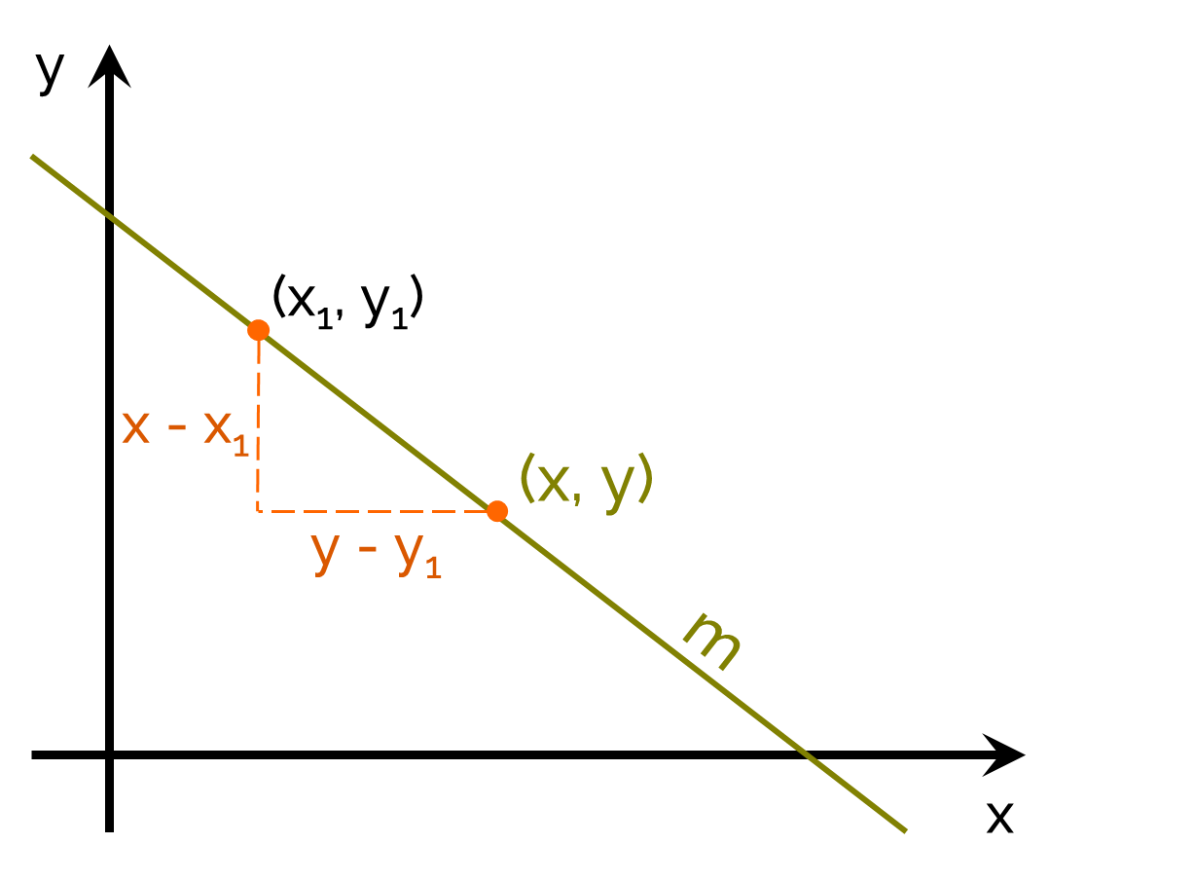# Point Slope Form Calculator

The point-slope form calculator will show you how to find the equation of a line from a point on that line and the line's slope.Point coordinates

Slope

## What is a slope?

Let's start with the basics. What is the slope? The slope, also known as the gradient, is the marker of a line's steepness. If it's positive, it means the line rises. If it's negative - the line decreases. If it's equal to zero, the line is horizontal.

You can find the slope between two points by estimating rise over run - the difference in height over a distance between two points.So, slope formula is:

`m = change in y / change in x = (y - y₁) / (x - x₁)`

The point-slope form equation is a rearranged slope equation.

To find the gradient of non-linear functions, you can use the average rate of change calculator.